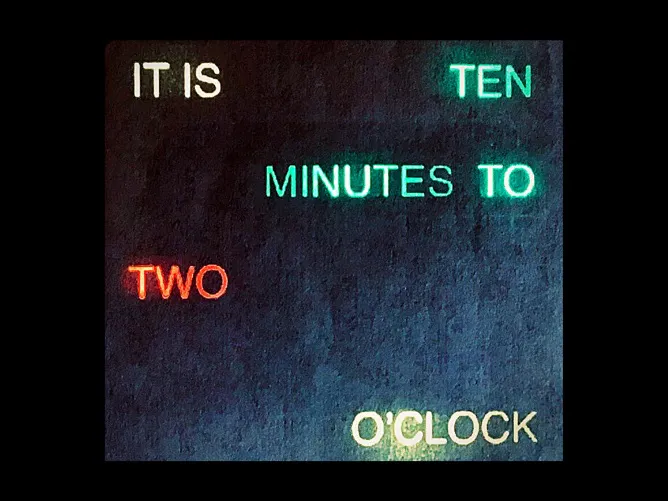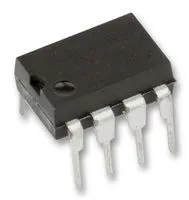# Minimal parts simple word clock

Simple word clock using 2812b leds.

BeginnerFull instructions provided1,495## Things used in this project

### Hardware componentsArduino Nano R3
×1Real Time Clock (RTC) DS 1307
×1
×22×1Jumper wires (generic)
×1
 Card stock, glue, misc supplies
×1

### Software apps and online servicesArduino IDE

### Hand tools and fabrication machinesSoldering iron (generic)

## Code

### wordclock2812.ino

Arduino
```#include <Wire.h>
#include "RTClib.h"
#include <FastLED.h>
#define NUM_LEDS 22
#define DATA_PIN 7
CRGB leds[NUM_LEDS];

RTC_DS1307 rtc;

void setup () {

Serial.begin(9600);
if (! rtc.begin()) {
Serial.println("Couldn't find RTC");
while (1);
}

if (! rtc.isrunning()) {
Serial.println("RTC is NOT running!");

}

{

}

}
void loop () {
DateTime now = rtc.now();
int h;

Serial.print(now.hour(), DEC);
h=(now.hour());
if (now.minute()>34){
h=h+1;
}

Serial.print(':');
Serial.print(now.minute(), DEC);
leds = CRGB::Yellow;
leds = CRGB::Yellow;
FastLED.show();
if (now.minute() <=4){
Serial.print ("nothing");

leds = CRGB::Black;
leds = CRGB::Black;
leds = CRGB::Black;
leds = CRGB::Black;
leds = CRGB::Black;
leds = CRGB::Black;
leds = CRGB::Black;

FastLED.show();
}
else if (now.minute() <=9){
Serial.print ("five minutes past");
leds = CRGB::Red;
leds = CRGB::Red;
leds = CRGB::Red;
FastLED.show();
}
else if (now.minute() <=14){
Serial.print ("ten minutes past");
leds = CRGB::Black;
leds = CRGB::Red;
leds = CRGB::Red;
leds = CRGB::Red;
FastLED.show();
}
else if (now.minute() <=19){
Serial.print ("quarter past");
leds = CRGB::Black;
leds = CRGB::Red;
leds = CRGB::Black;
leds = CRGB::Red;
FastLED.show();
}
else if (now.minute() <=24){
Serial.print ("twenty minutes past");
leds = CRGB::Black;
leds = CRGB::Red;
leds = CRGB::Red;
leds = CRGB::Red;
FastLED.show();
}
else if (now.minute() <=29){
Serial.print ("twenty-five minutes past");

leds = CRGB::Red;
leds = CRGB::Red;
leds = CRGB::Red;
leds = CRGB::Red;
FastLED.show();

}
else if (now.minute() <=34){
Serial.print ("half past");
leds = CRGB::Black;
leds = CRGB::Black;
leds = CRGB::Red;
leds = CRGB::Black;
leds = CRGB::Red;
FastLED.show();

}
else if (now.minute() <=39){
Serial.print ("twenty-five minutes to");
leds = CRGB::Black;
leds = CRGB::Black;
leds = CRGB::Red;
leds = CRGB::Red;
leds = CRGB::Red;
leds = CRGB::Red;
FastLED.show();

}
else if (now.minute() <=44){
Serial.print ("twenty minutes to");
leds = CRGB::Black;
leds = CRGB::Black;
leds = CRGB::Red;
leds = CRGB::Red;
leds = CRGB::Red;
FastLED.show();
}
else if (now.minute() <=49){
Serial.print ("quarter to");
leds = CRGB::Black;
leds = CRGB::Red;
leds = CRGB::Black;
leds = CRGB::Red;
FastLED.show();

}
else if (now.minute() <=54){
Serial.print ("ten minutes to");
leds = CRGB::Black;
leds = CRGB::Red;
leds = CRGB::Red;
leds = CRGB::Red;
FastLED.show();

}
else if (now.minute() <=59){
Serial.print ("five minutes to");
leds = CRGB::Black;
leds = CRGB::Red;
leds = CRGB::Red;
leds = CRGB::Red;
FastLED.show();
}

if (h==1){
leds = CRGB::Black;
leds = CRGB::Blue;
FastLED.show();
}
if (h==2){
leds = CRGB::Black;
leds = CRGB::Blue;
FastLED.show();
}
if (h==3){
leds = CRGB::Black;
leds = CRGB::Blue;
FastLED.show();
}
if (h==4){
leds = CRGB::Black;
leds = CRGB::Red;
FastLED.show();
}
if (h==5){
leds = CRGB::Black;
leds = CRGB::Red;
FastLED.show();
}
if (h==6){
leds = CRGB::Black;
leds = CRGB::Red;
FastLED.show();
}
if (h==7){
leds = CRGB::Black;
leds = CRGB::Blue;
FastLED.show();
}
if (h==8){
leds = CRGB::Black;
leds = CRGB::Blue;
FastLED.show();
}
if (h==9){
leds = CRGB::Black;
leds = CRGB::Blue;
FastLED.show();
}
if (h==10){
leds = CRGB::Black;
leds = CRGB::Blue;
FastLED.show();
}
if (h==11){
leds = CRGB::Black;
leds = CRGB::Blue;
FastLED.show();
}
if (h==12){
leds = CRGB::Black;
leds = CRGB::Green;
FastLED.show();
}

if (h==13){
leds = CRGB::Black;
leds = CRGB::Green;
FastLED.show();
}
if (h==14){
leds = CRGB::Black;
leds = CRGB::Green;
FastLED.show();
}
if (h==15){
leds = CRGB::Black;
leds = CRGB::Green;
FastLED.show();
}
if (h==16){
leds = CRGB::Black;
leds = CRGB::Green;
FastLED.show();
}
if (h==17){
leds = CRGB::Black;
leds = CRGB::Green;
FastLED.show();
}
if (h==18){
leds = CRGB::Black;
leds = CRGB::Green;
FastLED.show();
}
if (h==19){
leds = CRGB::Black;
leds = CRGB::Green;
FastLED.show();
}
if (h==20){
leds = CRGB::Black;
leds = CRGB::Green;
FastLED.show();
}
if (h==21){
leds = CRGB::Black;
leds = CRGB::Green;
FastLED.show();
}
if (h==22){
leds = CRGB::Black;
leds = CRGB::Green;
FastLED.show();
}
if (h==23){
leds = CRGB::Black;
leds = CRGB::Green;
FastLED.show();
}
if (h==0){
leds = CRGB::Black;
leds = CRGB::Red;
FastLED.show();
}

Serial.print(':');
Serial.print(now.second(), DEC);
Serial.println();

Serial.println();
delay(3000);
}
```

## Credits

### garysat

4 projects • 24 followers